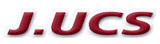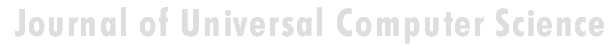Search
 Submission Procedure share: |available in:   PDF (50 kB) PS (35 kB)

get:
 Similar Docs BibTeX Write a comment
get:

DOI:   10.3217/jucs-001-01-0002

# High-radix Division with Approximate Quotient-digit Estimation

Peter Fenwick
Department of Computer Science, The University of
Auckland, Private Bag 92019, Auckland, New Zealand
p_fenwick@cs.auckland.ac.nz

Abstract: High-radix division, developing several quotient bits per clock, is usually limited by the difficulty of generating accurate high-radix quotient digits. This paper describes techniques which allow quotient digits to be inaccurate, but then refine the result. We thereby obtain dividers with slightly reduced performance, but with much simplified logic. For example, a nominal radix-64 divider can generate an average of 4.5 to 5.5 quotient bits per cycle with quite simple digit estimation logic. The paper investigates the technique for radices of 8, 16, 64 and 256, including various qualities of digit estimation, and operation with restricted sets of divisor multiples.

Keywords: Division, high radix, approximate digit estimates

Category: B2

# 1 Introduction

Division has always been one of the more difficult of the fundamental operations in computers. Some very early computers omitted it altogether, even if they included multiplication, relying on one of the multiplicative algorithms mentioned later. (Many modern super-computers also follow this well-established historical precedent!) Division was often restricted to basic restoring or non-restoring methods which develop only one bit per cycle, even on computers where multiplication handled two or more bits per cycle.

There are several methods for achieving binary division which is faster than the simple non-restoring method.

1. The basic techniques of efficient digit-by-digit binary division, originally using multiples of {-1, 0, +1}, were established by [Robertson 1958] and [Tocher 1956], summarised by [MacSorley 1961] and analysed by [Freiman 1961], who introduced the term SRT division. Robertson discusses Radix-4 SRT division and [Atkins 1970] extends the analysis to higher radix dividers (radices of 16, 64 and 256). [Wilson and Ledley 1961] describe division with shifting over 0s and 1s. A good discussion of these early methods is also given by [Flores 1963]. [Waser and Flynn 1982] describe these as subtractive algorithms because they are based on subtraction as the iterative operator.

Page 2

2. The development of very fast combinational multipliers [Wallace 1964] renewed interest in the older multiplication-intensive methods based on Newton-Raphson or Taylor series approximations to the reciprocal of the divisor. In most cases these methods provide quadratic convergence to the final value, doubling the number of accurate digits at each iteration. [Knuth 1969] (p244) presents a cubically convergent method which triples the accuracy at each step, but it requires more, less-convenient, arithmetic and is overall no faster than the simpler quadratic methods.) The repeated multiplications lead Waser and Flynn to describe these as multiplicative algorithms.

3. Other, very significant, developments are in some sense a melding of the subtractive and multiplicative methods. The basic iteration is still subtractive, but combinational multipliers are included as components to form divisor multiples and, often, quotient digits. For example, Byte Division, described by Waser and Flynn uses a ROM to estimate the divisor reciprocal which is then combined with the residue in a small combinational multiplier to estimate the quotient digit. With Booth recoding of the quotient digit a 4-input adder can form all 256 divisor multiples. Some improved methods of digit estimation are discussed by [Schwarz and Flynn 1993], and [Wong and Flynn 1992], who achieve speeds of at least 12 or 14 bits per iteration, and up to 53 bits per iteration. Following work by [Svoboda 1963], [Ercegovac, Lang and Montuschi 1993] have recently described a method based on pre-scaling the divisor and dividend which allows the development of 12 or more quotient bits per cycle. They again include multipliers as basic components. Thus while these are still essentially subtractive dividers, they use combinational multipliers to accomplish very high radix operation.

The work of this paper is in many ways a return to the traditional subtractive methods, but emphasises the combination of high-radix division (to minimise the time for a division) with relatively simple logic for quotient digit estimation (to minimise complexity and cost) and a minimal set of divisor multiples (again for complexity and cost). A unique feature is the trade-off between performance and complexity; without changing the basic design it is possible to reduce the available divisor multiples or the accuracy of the quotient digit estimation (or both) at the cost of only slightly slower division operation.

# 2 The Basic Principles of "Subtractive Division"

All of the subtractive methods depend on the relation below, as stated by Atkins 1961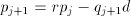Page 3

```where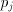= the residue used in the j-th cycle,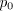= the initial dividend,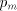= the remainder, and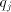= the j-th quotient digit

We also have that= the radix, eg 2, 4, 8, 16,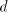= the divisor, and= the number of radix-r digits in the quotient
```

Verbally, we can note that we subtract a multiple () of the divisor from the residue and enter the sameas the corresponding quotient digit. By convention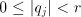, this ensuring that a properly chosen valuewill eliminate a digit ofand ensure that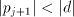and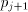is in the range to allow the iteration to proceed. The crux of most division methods lies in generating the correct value ofso that the residue is properly reduced and the generated digit is accurate. For high radices this may require considerable logic; so much so that Waser and Flynn consider that SRT algorithms are unsuitable for any radix greater than 4.

[Knuth 1969] (p235) shows that for any radix r, estimating the quotient digit by taking the two most-significant residue digits divided by one most-significant divisor digit will give an error of at most 2 in the estimate; he includes a refinement which ensures that the digit is usually exact, may be in error by 1, and never has an error of 2. The refinement is, however relatively expensive to implement and he does not discuss the necessary hardware. (It does however have interesting connections with the more recent techniques for producing very accurate quotient estimates.)

[Atkins 1970] presents an extensive analysis of SRT division and its extension to higher radices. He discusses redundancy of the quotient representation (for example, 3 may be represented as either 2+1 or 4-1) and states that With redundancy, the quotient digit need not be precise. Then from a detailed analysis of digitestimation logic, he shows that the number of bits to be examined is at least

Residue bits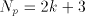or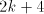, and

Divisor bits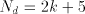where the radix r is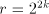```Radix      Residue      Divisor
bits         bits
4         5            7
8         6            8
16         7            9
64         9           11
256        11           13
```

Table 1. Atkins estimates ofbits to be examined

Page 4

For some typical radices, we find that the number of operand bits to be examined is as shown in [Tab. 1].

These results show that quotient estimation for high radix division is indeed a difficult process; even for radix 16 it is a function of at least 16 inputs. Atkins also discusses the problems of converting the quotient from the redundant code in which it is generated into the external binary form and states that a full-length quotient subtracter may be necessary. (This matter will considered later.)

A recent paper [Montuschi and Ciminiera 1994] considers the use of overredundant digits, where the quotient digits may equal or exceed the division radix. In the present context their most important result is that the quotient-digit estimation logic may be simplified by allowing a wider range of quotient digits. Many of their comments (and the over-redundant digits) are germane to the present work, but here we also allow the quotient digits to be inaccurate.

# 3 The new approach

Underlying most of this paper is the observation that at any stage of the division with divisor d the 'residue' p and 'partial quotient' q represent a value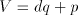. The value is unchanged if we put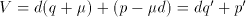. In other words we can add any quantity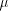to the quotient, provided that we also subtract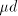from the residue. A quotient digit can be formed as a result of several operations with the same operand alignment; if the estimation logic gives a poor estimate of the quotient digit, we can 'hold' that division cycle and correct or refine the estimate until the residue is within range for the next reduction.

The new method involves placing a small low-precision adder at the low-order end of the quotient so that new digits are added into the quotient rather than jammed in as is usual. We also allow unshifted arithmetic to refine the quotient digit estimate, effectively holding the division at a particular stage until its result is satisfactory. We can use any multiple which we like, or any convenient combination of multiples, in constructing each quotient digit. In particular we do not insist that the generated quotient digit will immediately reduce the residue to the correct range, but are prepared to accept a poor estimate and then repair the damage from that estimate. There are two consequences -

• The bits entered into the quotient do not have to be exact. Small errors can be corrected by carry propagation within the quotient adder, provided that the carry is absorbed within the length of the adder. The divisor multiple need be accurate enough only to allow the residue to be driven toward zero at each step. The resulting changes to the quotient will be referred to as 'quotient adjustment'.

• If the chosen multiple estimate leaves the residue too far from zero, it is possible to hold the division at a step and subtract another divisor multiple without

Page 5

shifting. Thus if the logic estimated a multiple of 5 instead of the correct value of 6, a correction with a multiple of 1 will ensure the correct result. Carry propagation within the quotient adder will convert the initial estimate to the correct value. These will be referred to as 'correction cycles'.

Both aspects allow us to reduce the quality of quotient digit estimation without affecting the accuracy of the final result.

The second aspect is especially interesting. While it is relatively easy to provide logic which gives a good quotient estimate most of the time, it is much more difficult (and expensive) to generate an accurate value all of the time. (This complexity is evident from the results of Atkins, especially when compared with the look-up table sizes used here.) With a correction step available at any stage a bad estimate need not affect the final answer - it just requires a little longer to fix up. We can therefore trade off the complexity of the estimation logic against the overall division speed.

# 4 Limiting the quotient carry propagation.

An assumption of the present work is that the quotient has a short adder; a full-length quotient adder requires a considerable increase in logic complexity and should be avoided if possible. (This is exactly the situation discussed by Atkins and mentioned above in converting from redundantly-represented quotient digits.)

For a positive divisor, excessive quotient carry arises when the residue becomes negative after a subtraction and remains negative for a while thereafter. With conventional non-restoring division, the negative value will force a 0 quotient bit to be entered (from an unsuccessful subtraction). By comparison, the algorithm as described enters the multiple which was used (and was too large) and relies on a later negative digit to correct for the overdraw. If there is only a slight overdraw, the residue stays close to zero for several steps while zero quotient digits are generated and the carry must eventually propagate through all of these zero digits.

To minimise the quotient carry propagation, we monitor the sign of the result and, if it is negative, enter as a quotient digit the (multiple-1) and set a Qcarry flag; this is analogous to the action of simple non-restoring division. Qcarry is shifted in parallel with the quotient and added in on the next cycle. Thus we subtract 1 from the quotient, but add it back on the next cycle. The operation is similar during a correction cycle, except that the 1 is added directly into the quotient, without any shift.

To illustrate, consider a radix-8 divider where the residue goes just negative from a multiple of 6 and stays negative with no arithmetic (0 digit) for several cycles before being corrected with a -2 multiple.

Assuming that the simple algorithm generates the quotient digit sequence

Page 6

{ 6 0 0 -2 }, the generated quotient bits are { 110 000 000 } before the last digit and become the correct value { 101 111 111 110 } after the -2 multiple is added, but only after the carry propagates through 8 bits of the previous quotient.

With the Qcarry flag, we recognise the overdraw and enter an initial 5 instead of 6; this digit is now correct. On the next two cycles the generated digit of 0 is converted to -1 because of the negative residue, but the shifted Qcarry corresponds to an addition of 8, so the entered digit is 7 or bit pattern 111. We enter the correct quotient digit at each stage, avoiding lengthy carry propagation.

We may note that the Qcarry is in fact redundant, being identical to the residue sign. It is however convenient to regard it as a separate entity connected with the quotient rather than the residue. The carry from the main adder wraps-round into the quotient adder.

# 5 The complete division algorithm.

The final algorithm is shown in [Fig. 1], written in C but with some conditions in descriptive rather than explicit form. The function estDigit produces a suitable quotient digit estimate by some means (in the tests by a table lookup), including operations such as the limitation to complex multiples as described in [Section 11]. This program, and indeed all of the work in the paper, assumes a positive divisor.

```while (dividing)
{
Residue <<= BitsPerDigit;                       /* align residue */
Qdigit = estDigit (Residue, Divisor);                 /* estimate quot. digit */
Residue -= Qdigit * Divisor;                          /* adjust residue */
Quotient =
((Quotient + Qcarry) << BitsPerDigit) + Qdigit;
Qcarry = (Residue  <0);                            /* to stop long carries */
Quotient -= Qcarry;                                   /* and adjust quotient */

while (Residue_out_of_range)                          /* correction cycles */
{
Qdigit = estDigit (Residue, Divisor);              /* est. quot. digit */
Residue -= Qdigit * Divisor;                       /* adjust residue */
Quotient += Qdigit + Qcarry;                       /* adjust quotient */
Qcarry = (Residue < 0);
Quotient -= Qcarry;
}
}  /* end main divide loop */

if (Qcarry > 0) Quotient++;        /* assimilate quotient carry */
while (Residue < 0)             /* correction if -ve residue */
{ Residue += Divisor; Quotient--; }

```

Figure 1. The basic division program

Page 7

The first four lines of the main loop are essentially a standard high-radix division and are followed by two lines to control the quotient carry propagation. An inner loop handles the case of the residue being not reduced correctly, using code which is very similar to the main division code but without operand shifts. Finally, after the main loop is complete, we must assimilate any pending Qcarry and correct for a residue of the wrong sign.

A point which is not stated is that digit estimation in the inner, correction, loop must never give a zero digit because this loop must always change the residue; the digit must be forced to +1 or -1 depending on the sign of the residue.

# 6 The Hardware

The basic divider hardware is shown in [Fig. 2]. The differences from conventional division hardware are in the presence of the quotient adder and in the ability to operate in an unshifted mode during division. The quotient is shown with two paths from the quotient register, one shifted and one unshifted; the same applies to the residue register and main adder. Two shifts are wired into the residue and quotient logic; a shift of 3, 4, etc bits which determines the nominal radix of the operation and a zero shift which is used during correction cycles.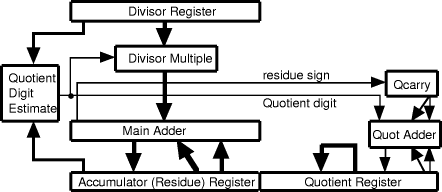Figure 2. Divider Hardware

In many cases the divisor multiple logic is limited to a 2-input adder/subtracter with shifters at each input up to the width of a quotient digit.

Page 8

The proposed algorithm was simulated by program using 32-bit integers and 64-bit long integers to provide a basic operand precision of 24 bits (48 bit dividend). In all cases the high-order bits of the divisor and residue are used to index a pre-computed table which yields the estimated multiple. The divisor is assumed normalised with its most-significant bit always 1. The initial tests are with radix-8 division (3 bits per cycle). The test cases were -

• A 7x7 table (7 residue bits and 7 divisor bits), which is similar in size to what Atkins predicts is needed for radix-8 division

• Three smaller sizes (6x6, 5x5, 4x4), the larger two of which are 'nearly good enough' for conventional division. The last is intended as a test of an economical estimation table.

• A table which examines only 3 residue bits and 3 divisor bits (just two significant divisor bits). This was tested as a minimal table which is easily implemented in combinational logic.

All cases were tested by a sequence of 100,000 divisions (the same sequence in all cases) counting the total add/subtract operations or cycles, the number of times that an earlier quotient was adjusted, and the number of additional correction cycles needed. With 8 octal digits to be developed for each 24-bit test operand, there are 8 'basic operations' for each test case, or 800,000 operations within each test.

The results are shown in [Tab. 2]. Each column heading shows first the radix and then the residue and divisor bits used to estimate the quotient digit for that test. The same heading convention will be followed for all of the results tables.

```                         8:7x7     8: 6x6     8: 5x5     8: 4x4      8:3x3
basic operations       800,000    800,000    800,000    800,000    800,000
quot. adjustments            0          0          0      1,292      9,412
adjustments (%)              0          0          0      0.158      1.085
correction cycles            0         19        699     16,395     66,862
corrections (%)              0      0.002      0.087      2.049      8.357
bits per cycle           3.000      3.000      2.997      2.940      2.769
performance              1.000      1.000      0.999      0.980      0.923
Quotient carry distance      0          1          2          6          6
```

Table 2. Radix-8 division, with varying look-up table sizes.

Small errors in the digit estimation show up in the 'quotient adjustments' which refine the prior quotient, but do not affect the residue or the speed. Larger errors manifest themselves as 'correction cycles' which modify the existing quotient and residue, and do slow the operation. In this table, the 'quotient adjustments' count only the adjustments which affect more than the least-significant quotient digit; we

Page 9

may expect every correction to alter this digit but count only those which spill into more significant digits. In no case does the quotient carry propagate over more than 2 digits (6 bits).

Whereas the largest, 7x7, table is able to predict a correct digit every time, the 6x6 table is inadequate by normal standards because it gives a few estimates which require correction. Even so it delivers a performance almost identical to the larger table (actually 2.99993 bits per cycle). The 5x5 and 4x4 tables are even less acceptable by normal criteria, with error rates of 0.1% and 2%, but here they still give performance within 0.1% and 2% of the optimal 3 bits per cycle. Even the minimal 3x3 table still yields the correct quotient digit 92% of the time and needs a correction cycle on only 8% of the steps, yielding nearly 2.77 bits per cycle.

Comparative results for different radices are given later in [Fig. 3] (showing bits per cycle) and in [Fig. 4] (showing relative performance). These figures include all of the significant and useful cases to be discussed and should be consulted for quick comparisons.

# 8 Effect of table aspect ratio

Although Knuth implies that it is better to examine more residue digits than divisor digits, Atkins shows in his analysis that it is desirable to examine about the same number of bits from each value, or perhaps a few more bits from the divisor. We next examine the effect of trading off residue bits against divisor bits, in all cases keeping constant the total number of tested bits. The results of [Tab. 3] are for the '5x5' table of the previous section, but similar results were obtained for other configurations.

For the present situation, where we are concerned only with obtaining a good estimate rather than the accurate value, it seems best to consider about the same number of bits from the two operands. Where the total number of bits is odd, the extra bit should be allocated to the residue. Results for the rest of the paper will mostly assume a 'square' table, without further justification.

```                                 8:6x4    8: 5x5    8: 4x6
basic operations               800,000   800,000   800,000
correction cycles                6,844       699     5,486
corrections (%)                  0.856     0.087     0.685
bits per cycle                   2.975     2.997     2.980
performance                      0.992     0.999     0.993
Quotient carry distance              6         2         3
```

Table 3. Radix-8 division, varying table aspect ratio.

Page 10

# 9 Radix-16 division (4 bits per cycle)

We repeat the above work for radix-16 division. Again, it is not difficult to get close to the ideal performance of 4 quotient bits per cycle. We now show four look-up tables, examining 7, 6, 5 and 4 bits of the residue and divisor. Atkins requires a 7x9 table for radix 16, which is larger than even the largest of the tables used here. The change in radix reduces the number of 'basic operations' to 600,000, (6 digits for a 24 bit operand) compared with 800,000 for radix-8 operation (8 digits for 24 bits).

Once again the larger tables give very nearly the maximum performance, with the 5x5 table within 1.5% of the ideal and even the 4x4 table (which considers only a single digit of each operand) only 8% off the possible speed. Quotient adjustments are again needed for the smaller tables, but even the smallest table never modifies more than 8 quotient bits (the current digit and its predecessor).

```                          16: 7x7    16: 6x6    16: 5x5     16: 4x4
basic operations          600,000    600,000    600,000     600,000
quot. adjustments               0          0        250       2,854
adjustments (%)                 0          0      0.041       0.442
correction cycles              57        615      8,489      46,507
corrections (%)             0.009       0.102     1.415       7.751
bits per cycle              4.000       3.996     3.944       3.712
performance                 1.000       0.999     0.986       0.928
Quotient carry distance         1           3         7           8
```

Table 4. Radix-16 division, with varying look-up table sizes.

For division with higher radices, we initially assume that all multiples are available and observe the effect of only the reduced digit-estimation logic. Actually not much extra hardware is needed to handle radix-64 and even radix-256 division - we certainly do not need an adder input for each possible power of two. By using Booth recoding of the quotient digit we can handle radix-64 with a 3-input adder for the divisor multiples and radix-256 with a 4-input adder. Later we reduce the range of multiples to allow simplified divisor-multiple generation logic. We retain the earlier sizes of look-up table as covering a reasonable range of practical sizes.

Although we assume the full range of divisor multiples for radix-64, we retain estimation logic which is quite small compared with Atkins predictions (9x 11 for radix 64). Particularly in the first two cases, 7x7 and 6x6 tables, we see from [Tab. 5] that the new algorithm largely absorbs any deficiencies of the digit estimation. The performance deteriorates markedly for the 5x5 and 4x4 tables, to the point where these are probably not worth considering, in comparison with the restricted

Page 11

cases later.

```                         64: 7x7    64: 6x6    64: 5x5     64: 4x4
basic operations         400,000    400,000    400,000     400,000
quot. adjustments              6        490       1746           0
adjustments (%)                0      0.114      0.330           0
correction cycles          3,492     29,744    128,667     382,634
corrections (%)            0.873      7.436     32.167      95.659
bits per cycle             5.948      5.585      4.540       3.067
performance                0.991      0.931      0.757       0.511
Quotient carry distance        9         12         11           6
```
Table 5. Radix-64 division - full multiples.

Repeating the exercise for radix-256, we obtain the results of [Tab. 6]. The relatively poor quality of the digit estimates is even more noticeable here, but even so by examining just a single digit (8 bits) of the residue and divisor we achieve nearly 7.5 bits per cycle.

```                        256: 8x8    256: 8x7    256: 7x7    256: 6x6    256: 5x5
basic operations         300,000     300,000     300,000     300,000     300,000
quot. adjustments             64         558         276           0           0
adjustments (%)            0.019       0.157       0.071           0           0
correction cycles         20,247      56,402      90,815     288,049     882,365
corrections (%)            6.749       18.80       30.27       96.02       294.1
bits per cycle             7.494       6.734       6.141       4.081       2.030
performance                0.937       0.842       0.768       0.510       0.254
Quotient carry distance       13          16          14           8           8
```
Table 6. Radix-256 division - full multiples.

By comparing this table with the previous one, we see that the performance is largely determined by the unexamined bits of the most-significant digit. Thus examining a complete digit (6 or 8 bits respectively for radix-64 and radix-256) gives about 93% of the ideal performance, one bit less (5 or 7 bits) gives 75% and 2 bits less 51%. Nevertheless, it is interesting that reasonable performance is still possible if the estimation logic examines only part of the most-significant digits of the residue and divisor. As a more general observation, the algorithm is robust with respect to changes in the digit prediction logic. A poor prediction does not impair the final result, but may delay achieving that result.

Practically though, it is clear that operation with a radix of 256 is not really satisfactory, at least with the size of look-up table which is used. With a 7x7 table, the performance is very little better than that of a radix-64 divider (6.14 bits, compared with 5.95). The benefit of the higher radix barely offsets the penalty of examining partial digits.

Page 12

# 11 Division with few multiples available.

Divisor multiples can be divided into 3 categories -

• 'Shifted values', available by just shifting left the raw value (1, 2, 4, 8,),
• 'Simple multiples', being the sum or difference of pairs of shifted values (3, 5, 6, 7, 9,),
• 'Complex multiples', which require the combination of 3 or more shifted values (11, 13,).
In this section we examine the performance if the only available multiples are the 'shifted values' and the 'simple multiples'. We assume that a 'large' shift is available (e g 6 places for radix-64). The initial operation on the shifted residue will be followed in many cases by 'corrections' on the unshifted residue as we simulate the more difficult multiples or quotient digits.

```                         16 7x7      16 6x6      16 5x5     16 4x4
basic operations        600,000     600,000     600,000    600,000
quot. adjustments             0           0         174      4,124
adjustments (%)               0           0       0.027      0.604
correction cycles        22,151      22,725      34,207     82,868
corrections (%)           3.692       3.788       5.701     13.811
bits per cycle            3.858       3.854       3.784      3.515
performance               0.964       0.964       0.946      0.879
Quotient carry distance       2           2           5          8
```

Table 7. Radix-16 division, with limitation to 'simple' multiples.

Initially we examine radix-16, even though a 2-input adder is adequate to form all of the multiples with Booth recoding of the quotient digits. The estimation tables do not use Booth recoding but are just recoded versions of the previous ones with, for example, 11 being rounded to 10 or 12.

As expected, there is some performance degradation as compared with the previous case where all multiples were assumed to be available. However even the simplest case still delivers over 3.5 bits per cycle. The speed is better than we would expect from noting that 1/8 of the multiples are unavailable and must be simulated; about half of these cases are just absorbed into the general operation and do not require explicit correction cycles.

For higher radices it is especially useful to avoid a complete suite of divisor multiples. We still restrict ourselves to simple multiples of the form.

Page 13

```
64: 7x7     64: 6x6     64: 5x5     64: 4x4
basic operations         400,000     400,000     400,000     400,000
quot. adjustments             10         736       1,482           0
adjustments (%)            0.002       0.143       0.250           0
correction cycles         91,476     116,488     193,466     412,402
corrections (%)            22.87       29.12       48.37       103.1
bits per cycle             4.883       4.647       4.044       2.954
performance                0.814       0.775       0.674       0.492
Quotient carry distance        6          12          11           6

single corrections        91,448     111,560     140,974     147,143
double corrections            14       2,464      26,246      85,618
> double corrections           0           0           0      30,116
```
Table 8. Radix-64 division, with limitation to simple multiples.
```                        256: 8x8     256: 7x7     256: 6x6
basic operations         300,000      300,000      300,000
correction cycles        187,415      245,222      399,179
corrections (%)            62.47        81.74        133.1
bits per cycle             4.924        4.402        3.433
performance                0.616        0.550        0.429
Quotient carry distance        9            9            8

single corrections       173,975       152,214     112,568
double corrections         6,810        46,360      85,170
> double corrections           0            96      36,544
```
Table 9. Radix-256 division, with limitation to simple multiples.

The results in [Tab. 8] and [Tab. 9] are extended to show details of the corrections. Radix-64 division is quite successful, generating an average of nearly 5 bits per cycle with either of the two larger tables. However the smallest table (4x4) is actually inferior to radix-16 with the same table size. The radix-256 results are inferior to the radix-64 results, showing the effect of having relatively fewer multiples available and having to rely much more on correction cycles.

With radix-16, restricted multiples cover 7/8 or 87.5% of the total range of multiples. With radix-64 only 33 multiples are available (51.6% coverage), but only a single correction cycle is ever needed in most cases. Radix-256 uses 58 multiples (22.6%) and the sparse coverage requires many more correction cycles even with the larger tables. (Both radix-64 and radix-256 actually use occasional multiples of 66, 68, 258

Page 14

and 260, which are available without penalty or extra hardware given that 64 and 256 are provided.) While radix-64 operation is acceptable, there is clearly no benefit in using this method for a radix-256 divider.

# 12 The effect of using only shifted values as multiples

As an extreme restriction on the available multiples, we can restrict them to just powers of 2 (those which are available by shifting). We retain the original style of lookup tables, even though they are clearly inappropriate in this case; we should be able to achieve comparable performance with much simpler quotient estimation.

```
64: 7x7    64: 6x6     64: 5x5     256:7x7
basic operations        400,000    400,000     400,000     300,000
quot. adjustments             0          0           0           0
adjustments (%)               0          0           0           0
corrections             407,228    428,667    484,1 62     554,306
corrections (%)           101.8      107.2       121.0       184.8
bits per cycle            2.973      2.896       2.714       2.809
performance               0.496      0.483       0.452       0.351
Quotient carry distance       5          5           5           9
```

The division should tend to be equivalent to a variable shift length algorithm, with multiples of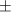1, shifting over strings of 0s and 1s. Variable shift algorithms are known to have an asymptotic performance of about 3 bits per cycle (2.5 - 3.5, see [Flores 1963]), a performance which is confirmed here in [Tab. 10]. As for the previous high radix operations, the performance is better for a radix of 64 than for 256.

# 13 Graphical summary of results

In [Fig. 3] and [Fig. 4] we show the performance results for the various radices and sizes of look-up table. Figure 3 shows the average quotient bits delivered per cycle, while Figure 4 shows the performance for each radix, with 100% being N bits/cycle for a radix of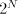. Results are shown for only the more realistic cases of full multiples and restricted multiples. Results for corrections and quotient adjustments are not presented; they are essentially internal or intermediary phenomena whose consequences are apparent in the final performance.

Page 15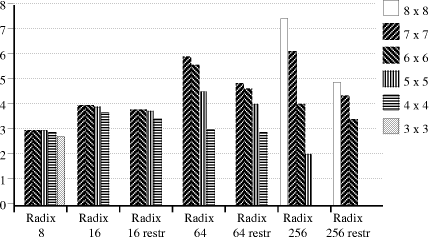Figure 3. Average bits/cycle, for different radices and table sizes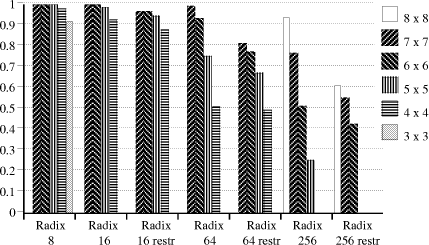Figure 4. Relative performance, for different radices and table sizes

The graphs show quite clearly that a table should work with about 1 digit for each input; a 6x6 table for example gives negligible benefit on a radix-16 divider, but with radix-256 gives fewer bits per cycle that with radix-64. This will be mentioned again later, in connection with some other recent work.

# 14 Generation of the quotient digits

The present paper has generated quotient digits only from look up tables addressed

Page 16

by the high order digits of the residue and divisor. This technique might not be the best one and in that regard the current work should be regarded more as a feasibility study of the new technique.

The usual problem in division is that the critical path consists of the generation of the quotient digit, then the generation of the corresponding divisor multiple and finally the addition/subtraction, in other words all of the difficult operations! [Montuschi and Ciminiera 1994] point out indeed that many methods of 'improving' division really do little more than move the dominant delay around the critical path! Nevertheless there are several ways of accelerating the digit estimation.

1. The 'divisor pre-scaling' methods of [Ercegovac, Lang and Montuschi 1993] and [Svoboda 1963] trivialise the generation of the quotient digit, but at the cost of preliminary arithmetic to get the divisor into a suitable form. With a normalised divisor, they subtract appropriately shifted multiples of the divisor from itself to produce a divisor of the form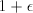, where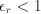. At the same time they apply similar transformations to the dividend; effectively they multiply the divisor and dividend by the same factor, leaving the quotient unchanged. With the divisor of that form, the high order digits of the residue are precisely the desired quotient digit. Without recoding the divisor digits we may expact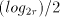iterations to reduce the divisor and the same number of parallel operations to transform the dividend, giving a total of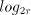preliminary operations. The cost of these operations would have to be offset against the reduction in the cycle time from the improvement in the time to generate quotient digits. A problem is that it does not yield a remainder.

2. [Wong and Flynn 1992] present quotient digit logic which operates in parallel with the other operations and allows much more overlap and correspondingly higher speed. Their logic does however assume the correctness of the previous quitient digit, using that digit as part of the input for the current estimate. The complexities of handling an approximate previous digit may make their full method unwieldy in this case. However, the essence of the method, which derives the actual logical functions of the quotient bits as explicit functions of the other bits, may still be appropriate.

3. The quotient digit may be estimated from the difference of the logarithms of the divisor and residue (or their high order bits). Although this will still require look-up tables or equivalent logic to produce the logarithms, the table sizes are now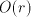, rather than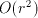. Conversion from the difference back to the actual digit estimate will require logic of similar scale to produce the antilog. Depending upon the logic family, the complex of 3 small tables (or equivalent) will certainly be simpler and may be faster than the single large lookup table. Note that we do not require wholly accurate digit estimates.

Operation with logarithms has been tested and verified, with similar results to the direct table lookup. The logarithm, if in base 2, needs as many fractional

Page 17

bits as there are bits in the radix, and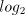integral bits. Thus radix-16 requires a logarithm of the form xx.xxxx, and radix-256 of the form xxx.xxxxxxxx. The detailed results are slightly different from those for the direct look-up tables, even though the formulae are nominally equivalent. In some cases there is a slight degradation in performance but many cases improve by 1 - 2%. This demonstrates both the robustness of the algorithm with respect to slight changes in the digit estimates and the possibility of improving the performance in particular cases by fine tuning the digit estimation.

4. An interesting possibility, which has not been investigated, might be to use hybrid analogue/digital techniques in the quotient digit estimation. Similar methods have been used in a recent adder design [Kawahito et al 1994]. The essential point is that the algorithms described here can accomodate poor quotient digit estimates without undue penalty. If hybrid techniques can achieve useful simplification while delivering reasonably good estimates, they could be worth consideration.

It is not easy to predict which is the best method. Most of the table-lookup methods insert extra delay into the division cycle and slow down every cycle; they may be better if there is spare time in each cycle. The prescaling methods however allow faster cycles within the division proper, but require additional steps for the preliminary adjustments and may be better where the system clock matches the critical path delay within the division logic.

# 15 Other recent work

A very recent paper (published since this paper was submitted) presents some very similar techniques [Cortadella and Lang, 1994]. A comparison of the two methods is conveniently presented as a series of points (with references to 'this paper' and 'their paper' having the obvious meanings).

• Even though the techniques are similar, the underlying philosophies are different. This paper works in terms of an approximation to the exact quotient digit, with possible refinements to that estimate. Their paper emphasises the speculative nature of the digit estimation, with options such withdrawing the estimation completely or accepting only some of the estimated bits.

• This paper always develops a fixed number of bits, perhaps taking several cycles to achieve an accurate digit. Their paper however allows a 'partial advance' to develop fewer bits on some cycles, accepting only as many quotient bits as are known to be accurate. Thus both approaches may develop fewer that the nominal bits per cycle, but in different ways. (Their 'basic scheme', without partial advance is similar toe the ideas of this paper.)

Page 18

• This paper places considerable emphasis on developing the quotient by adding in each new digit. Although perhaps not really apparent from this paper this was the original idea which led to the work, but had to be supplemented by allowing some digits to require several cycles. Their paper acknowledges a quotient adjustment by1, but does not explore the consequences of possible quotient carry propagation.

• Their paper goes to some trouble to develop a good 'speculation function' equivalent to the 'estimation tables' used in this paper. This paper (while assuming look-up tables for quotient-digit estimation) provides a much more comprehensive treatment of the effects of varying the table size and therefore the accuracy of the quotient digit.

• One of their optimisation options is to reduce the number of outputs from the speculation function. This parallels exactly the use of the 'simple multiples' in this paper.

• Their paper has much more detail concerning the hardware consequences, costs and physical aspects of their design.

• Their results on there being an optimal complexity of the digit-estimation logic are supported by the results here which show, for example, a 6x6 table giving its best performance with radix-64 division.

• Both papers rely very heavily on computer simulations of algorithms which are largely unpredictable in internal details, even though the final result is very well determined.

The two papers present different approaches to the same problem, both illustrating that high-radix division does not require exact high precision quotient-digit estimates.

We have shown that it is possible to obtain satisfactory high-radix division, even when the division process is subject to one of two significant restrictions -

1. Limited precision digit estimation. By allowing the quotient logic to assimilate corrections from later digits, and by allowing an occasional 'hold' of the division process, it is possible to estimate quotient digits to quite low precision. The corrections are needed in relatively few cases and lead to quite small performance degradation except for extremely simple estimation logic. For example, a divider which examines just a single digit of the residue and divisor (i e 3 or 4 bits of each) can average over 2.75 bits per cycle for radix-8 division and 3.7 bits per cycle for radix-16 division.

2. Limited divisor multiples. High radix division is expensive in its logic to

Page 19

estimate quotient digits and its logic to form a complete suite of divisor multiples. We have demonstrated that a radix-64 divider (generating a nominal 6 bits per cycle) can generate an average of more than 4.5 bits per cycle using only those multiples which can be formed with a two input adder-subtracter and considering only a single 6-bit digit of each of the residue and divisor.

The tests indicate that the new techniques are appropriate for radices up to 64, but less satisfactory with a radix of 256, largely because of restrictions on the digit-estimation logic and the number of divisor multiples which are available.

If we have a multiplier which is designed for, say, radix-64 multiplication, its hardware can be used as the basis of a divider with nominal radix-64 operation. This paper shows that, with relatively simple digit estimation logic, it is easy to achieve, if not the full 6 bits per cycle, then certainly 4 or 5 bits.

The lookup tables are generated as a simple function of the high-order residue and divisor bits, assumed to index the rows and columns respectively of the table. The process is parametrised to facilitate the generation of tables of differing sizes and shapes, and for differing radices. The tables are generated for positive residue and divisor (with the most-significant divisor bit always 1) and the negative half then generated as the complement of the positive half. There is evidence that minor improvements might be possible by fine-tuning some table entries in particular cases but that has mot been attempted.

The basic parameters are

ResBits
the number of residue bits to examine (excluding the sign)
DvsBits
the number of divisor bits to examind, including the normalised bit
the radix of the quotient digit digit

From these are derived several other values

DigitBits (Radix = 1 << DigitBits)
the bits to represent a digit
maxRow (1 << ResBits)
the maximum row of the table
maxCol (1 << DvsBits)
the maximum column of the table
minCol (maxCol / 2)
the minimum column of the table
beta (2 << DigitBits)*minCol/maxRow
a scaling factor

Logically, the table rows extend from -maxRow to maxRow - 1, and the columns from minCol to maxCol - 1. We calculate also a scaling factor chosen to give an intermediate result of twice the true estimate. A mapping table then rounds the intermediate result of twice the true estimate. A mapping table then rounds the intermediate value to the correct estimate, or rounds with suppression of complex multiples.

Page 20

The essential loops for producing the table are then

```for (col = minCol; col <= maxCol; col++)
for (row = 0; row <= maxRow; row++)
Table[row + maxRow][col - minCol] = (beta * row) / col;
```

For operation with less than the full set of divisor multiples the table values are rounded to the nearest available multiple.

# References

[Atkins 1970] D.E. Atkins, "Higher-Radix Division Using Estimates of the Divisor and Partial Remainders", IEEE Trans. Comp., August 1970, pp 720-733

[Cortadella and Lang, 1994] J. Cortadella and T. Lang, "High-Radix Division and Square Root with Speculation", IEEE Trans. Comp., August 1994, pp 919-931

[Ercegovac, Lang and Montuschi 1993] M.D. Ercegovac, T. Lang, P. Montuschi, "Very High Radix Division with Selection by Rounding and Prescaling", Proc. Eleventh IEEE Symp. Comp. Arithmetic, 1993, pp 112-119

[Freiman 1961] C.V. Freiman, "Statistical Analysis of Certain Binary Division Algorithms", Proc. IRE, Vol 49, No 1, Jan 1961, pp 91-103

[Hwang 1979] K. Hwang, "Computer Arithmetic: principles, architecture and design", John Wiley and Sons, New York, 1979

[Flores 1963] Ivan Flores, "The Logic of Computer Arithmetic", Prentice-Hall, Englewood Cliffs, 1963

[Kawahito et al 1994] S. Kawahito, M. Ishida, T. Nakamura, M. Kamyama, T. Higuchi, "High-Speed Area-Efficient Multiplier Design Using Multiple-Valued Current-Mode Circuits", IEEE Trans Comp., Vol 43, No 1, Jan 1994, pp 34-42

[Knuth 1969] D.E. Knuth,"The Art of Computer Programming", Vol 2 Seminumerical Algorithms, Addison Wesley 1969

[MacSorley 1961] O.L. MacSorley,"High Speed Arithmetic in Binary Computers", Proc. IRE, Vol 49, Jan 1961, pp 67-91

[Montuschi and Ciminiera 1994] P. Montuschi and L. Ciminiera, "Over-Redundant Digit Sets and the Design of Digit-By-Digit Division Units", IEEE Trans. Comp., Vol 43, No 3, March 1994, pp 269-277

[Robertson 1958] J.E. Robertson, "A New Class of Digital Division Methods", IEEE Trans. Comp., Vol C-7, No 8, September 1958, pp 218-222

[Svoboda 1963] A. Svoboda, "An Algorithm for Division", Information Proc Machines, Vol 9, pp 25-32, 1963

Page 21

[Schwarz and Flynn 1993] E.M. Schwarz, M.J. Flynn, "Parallel High-Radix Nonrestoring Division", IEEE Trans. Comp., Vol 42, No 10, Oct 1993, pp 1234-1246

[Tocher 1956] K.D. Tocher, "Techniques of Multiplication and Division for Automatic Binary Computers", Q. J. Mech. Appl. Math. Vol 11 Pt 3 pp 364-384

[Wallace 1964] C.S. Wallace, "A Suggestion for a Fast Multiplier", IEEE Trans. Elec. Comp., Vol EC-13, Feb 1964, pp 14-17

[Waser and Flynn 1982] S. Waser, M.J. Flynn, "Introduction to Arithmetic for Digital Systems Designers", Holt, Reinhart and Winston New Yoerk, 1982

[Wilson and Ledley 1961] J.B. Wilson, R.S. Ledley,"An Algorithm for Rapid Binary Division", IRE Trans. Elec. Comp. Vol EC-10, Dec 1961, pp 662-670

[Wong and Flynn 1992] D. Wong, M. Flynn, "Fast Division Using Accurate Quotient Approximations to Reduce the Number of Iterations", IEEE Trans. Comp., Vol 41, No 8, Aug 1992, pp 981-995

Page 22